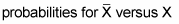##### Probability For DummiesIf you're going to take a probability exam, you can better your chances of acing the test by studying the following topics. They have a high probability of being on the exam.

• The relationship between mutually exclusive and independent events

• Identifying when a probability is a conditional probability in a word problem

• Probability concepts that go against your intuition

• Marginal, conditional, and joint probabilities for a two-way table

• The Central Limit Theorem:• When to use a permutation and when to use a combination

• Finding E(X) from scratch and interpreting it

• Sampling with replacement versus without replacement

• The Law of Total Probability and Bayes' Theorem

• When the Poisson and exponential are needed in the same problem